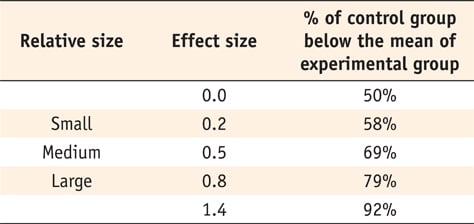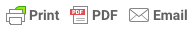# What does effect size tell you?

### What does effect size tell you?

By , published 2019

Statistical significance is the least interesting thing about the results. You should describe the results in terms of measures of magnitude – not just, does a treatment affect people, but how much does it affect them.

###### What is effect size?

Effect size is a quantitative measure of the magnitude of the experimenter effect. The larger the effect size the stronger the relationship between two variables.

You can look at the effect size when comparing any two groups to see how substantially different they are. Typically, research studies will comprise an experimental group and a control group. The experimental group may be an intervention or treatment which is expected to effect a specific outcome.

For example, we might want to know the effect of a therapy on treating depression. The effect size value will show us if the therapy as had a small, medium or large effect on depression.

###### How to calculate and interpret effect sizes

Effect sizes either measure the sizes of associations between variables or the sizes of differences between group means.

### Cohen's d

Cohen's d is an appropriate effect size for the comparison between two means. It can be used, for example, to accompany the reporting of t-test and ANOVA results. It is also widely used in meta-analysis.

To calculate the standardized mean difference between two groups, subtract the mean of one group from the other (M1 – M2) and divide the result by the standard deviation (SD) of the population from which the groups were sampled.A d of 1 indicates the two groups differ by 1 standard deviation, a d of 2 indicates they differ by 2 standard deviations, and so on. Standard deviations are equivalent to z-scores (1 standard deviation = 1 z-score).Cohen suggested that d = 0.2 be considered a 'small' effect size, 0.5 represents a 'medium' effect size and 0.8 a 'large' effect size. This means that if two groups' means don't differ by 0.2 standard deviations or more, the difference is trivial, even if it is statistically significant.

### Pearson r correlation

This parameter of effect size summarises the strength of the bivariate relationship. The value of the effect size of Pearson r correlation varies between -1 (a perfect negative correlation) to +1 (a perfect positive correlation).According to Cohen (1988, 1992), the effect size is low if the value of r varies around 0.1, medium if r varies around 0.3, and large if r varies more than 0.5.### The p-value is not enough

A lower p-value is sometimes interpreted as meaning there is a stronger relationship between two variables. However, statistical significance means that it is unlikely that the null hypothesis is true (less than 5%).

Therefore, a significant p-value tells us that an intervention works, whereas an effect size tells us how much it works.

It can be argued that emphasizing the size of effect promotes a more scientific approach, as unlike significance tests, effect size is independent of sample size.

### To compare the results of studies done in different settings

Unlike a p-value, effect sizes can be used to quantitatively compare the results of studies done in a different setting. It is widely used in meta-analysis.